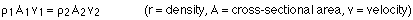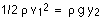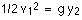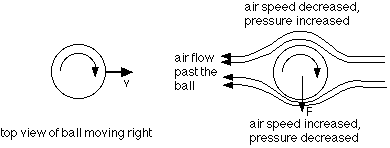## Fluid dynamics and Bernoulli's equation

11-10-99

Sections 10.7 - 10.9

### Moving fluids

Fluid dynamics is the study of how fluids behave when they're in motion. This can get very complicated, so we'll focus on one simple case, but we should briefly mention the different categories of fluid flow.

Fluids can flow steadily, or be turbulent. In steady flow, the fluid passing a given point maintains a steady velocity. For turbulent flow, the speed and or the direction of the flow varies. In steady flow, the motion can be represented with streamlines showing the direction the water flows in different areas. The density of the streamlines increases as the velocity increases.

Fluids can be compressible or incompressible. This is the big difference between liquids and gases, because liquids are generally incompressible, meaning that they don't change volume much in response to a pressure change; gases are compressible, and will change volume in response to a change in pressure.

Fluid can be viscous (pours slowly) or non-viscous (pours easily).

Fluid flow can be rotational or irrotational. Irrotational means it travels in straight lines; rotational means it swirls.

For most of the rest of the chapter, we'll focus on irrotational, incompressible, steady streamline non-viscous flow.

### The equation of continuity

The equation of continuity states that for an incompressible fluid flowing in a tube of varying cross-section, the mass flow rate is the same everywhere in the tube. The mass flow rate is simply the rate at which mass flows past a given point, so it's the total mass flowing past divided by the time interval. The equation of continuity can be reduced to:Generally, the density stays constant and then it's simply the flow rate (Av) that is constant.

### Making fluids flow

There are basically two ways to make fluid flow through a pipe. One way is to tilt the pipe so the flow is downhill, in which case gravitational kinetic energy is transformed to kinetic energy. The second way is to make the pressure at one end of the pipe larger than the pressure at the other end. A pressure difference is like a net force, producing acceleration of the fluid.

As long as the fluid flow is steady, and the fluid is non-viscous and incompressible, the flow can be looked at from an energy perspective. This is what Bernoulli's equation does, relating the pressure, velocity, and height of a fluid at one point to the same parameters at a second point. The equation is very useful, and can be used to explain such things as how airplanes fly, and how baseballs curve.

### Bernoulli's equation

The pressure, speed, and height (y) at two points in a steady-flowing, non-viscous, incompressible fluid are related by the equation:Some of these terms probably look familiar...the second term on each side looks something like kinetic energy, and the third term looks a lot like gravitational potential energy. If the equation was multiplied through by the volume, the density could be replaced by mass, and the pressure could be replaced by force x distance, which is work. Looked at in that way, the equation makes sense: the difference in pressure does work, which can be used to change the kinetic energy and/or the potential energy of the fluid.

### Pressure vs. speed

Bernoulli's equation has some surprising implications. For our first look at the equation, consider a fluid flowing through a horizontal pipe. The pipe is narrower at one spot than along the rest of the pipe. By applying the continuity equation, the velocity of the fluid is greater in the narrow section. Is the pressure higher or lower in the narrow section, where the velocity increases?

Your first inclination might be to say that where the velocity is greatest, the pressure is greatest, because if you stuck your hand in the flow where it's going fastest you'd feel a big force. The force does not come from the pressure there, however; it comes from your hand taking momentum away from the fluid.

The pipe is horizontal, so both points are at the same height. Bernoulli's equation can be simplified in this case to:The kinetic energy term on the right is larger than the kinetic energy term on the left, so for the equation to balance the pressure on the right must be smaller than the pressure on the left. It is this pressure difference, in fact, that causes the fluid to flow faster at the place where the pipe narrows.

### A geyser

Consider a geyser that shoots water 25 m into the air. How fast is the water traveling when it emerges from the ground? If the water originates in a chamber 35 m below the ground, what is the pressure there?

To figure out how fast the water is moving when it comes out of the ground, we could simply use conservation of energy, and set the potential energy of the water 25 m high equal to the kinetic energy the water has when it comes out of the ground. Another way to do it is to apply Bernoulli's equation, which amounts to the same thing as conservation of energy. Let's do it that way, just to convince ourselves that the methods are the same.

Bernoulli's equation says:But the pressure at the two points is the same; it's atmospheric pressure at both places. We can measure the potential energy from ground level, so the potential energy term goes away on the left side, and the kinetic energy term is zero on the right hand side. This reduces the equation to:The density cancels out, leaving:This is the same equation we would have found if we'd done it using the chapter 6 conservation of energy method, and canceled out the mass. Solving for velocity gives v = 22.1 m/s.

To determine the pressure 35 m below ground, which forces the water up, apply Bernoulli's equation, with point 1 being 35 m below ground, and point 2 being either at ground level, or 25 m above ground. Let's take point 2 to be 25 m above ground, which is 60 m above the chamber where the pressurized water is.We can take the velocity to be zero at both points (the acceleration occurs as the water rises up to ground level, coming from the difference between the chamber pressure and atmospheric pressure). The pressure on the right-hand side is atmospheric pressure, and if we measure heights from the level of the chamber, the height on the left side is zero, and on the right side is 60 m. This gives:### Why curveballs curve

Bernoulli's equation can be used to explain why curveballs curve. Let's say the ball is thrown so it spins. As air flows over the ball, the seams of the ball cause the air to slow down a little on one side and speed up a little on the other. The side where the air speed is higher has lower pressure, so the ball is deflected toward that side. To throw a curveball, the rotation of the ball should be around a vertical axis.It's a little more complicated than that, actually. Although the picture here shows nice streamline flow as the air moves left relative to the ball, in reality there is some turbulence. The air does exert a force down on the ball in the figure above, so the ball must exert an upward force on the air. This causes air that travels below the ball in the picture to move up and fill the space left by the ball as it moves by, which reduces drag on the ball.